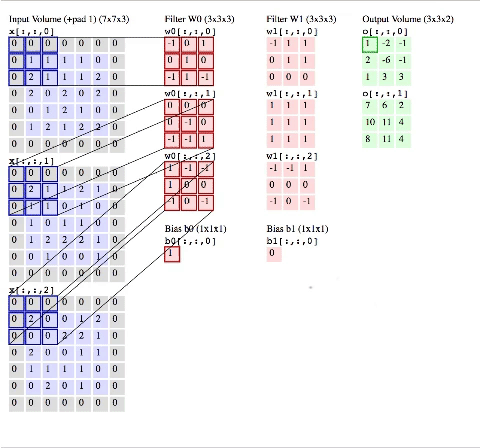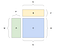# Demystifying Convolution in Popular Deep Learning Framework — Caffe

In this era of artificial intelligence, one might safely say that convolution is the heart of deep learning & computer vision. Several popular deep learning architectures, such as AlexNet, GoogleNet, ResNet, VGG, Inception, are using convolution as one of its computation building blocks. Convolution is being used to extract spatial information out of an image, in order to help them classify the image itself.

We won’t talk much detail on how the convolution works, but will rather explain on how convolution commonly being computed in a modern deep learning framework, or specifically, Caffe. We already have the working learning algorithm, the back-propagation of error. The challenge now is to make it run efficiently in today’s common hardwares, thus the importance of understanding how the convolution being calculated in a modern deep learning framework.

Before we continue further, I would suggest you to read a more comprehensive explanation about convolution here.Image by CS231n  How two-dimensional convolution works in producing the output tensor(s).

The illustration shows a two-dimensional convolution process. We take two-dimensional inputs, convolve two-dimensional filters around it, and produce two-dimensional outputs. Convolution process is an iterative process which takes sequence of steps, in order to compute all elements in the input layer.

As an example, we have an image, or tensor, with the width (W), height (H), and depth (D). We also have a patch called filter sized F in height & width and depth D. We apply K number of filters with that size to the input. The pseudo code as follows:

As seen above, there are several nested iterations in the process, or in other words, an intensive task for the CPU. This kind of task, when paired with a huge size of input tensor (which usually the case as we generally deals with high-resolution images), produces high latency of computation.

To alleviate such problem, several methods of optimisation has been introduced. For instance, we could change the computation perspective from an iterative method to a more “mathematically convenience” operation, like multiplication. By transforming the shape of input tensor and filters into matrices, we could turn the convolution iterative process into a ‘mere’ matrix to matrix multiplication.

In more popular term, a matrix to matrix multiplication is also known as general matrix-matrix multiplication, abbreviated as GEMM.

GEMM takes two matrices, then do a dot product over it (element-wise multiplication followed by addition). A M x P matrix multiplied by P x N matrix, produced M x N matrix as illustrated below.Matrix to Matrix Multiplication. An MxP matrix A multiplied by PxN matrix B produced MxN matrix C.

Now the next question is, “How do we transform and arrange all elements in our input and filters that we want to convolve, into GEMM matrices?”

Before we jump to the answer, let’s review all of the elements involved in convolution process. Several parameters which are important in convolution process are listed as follows:

• Wi : Input tensor width

Let’s construct a simple illustration to understand all terms mentioned above

In the illustration above, we have a 5x5 matrix as our input (Wi = 5 and Hi = 5). We add full zero padding to our input matrix with an offset of one (P = 1), enclosing all the side of our input. Our filters size are 3x3, thus the value of F = 3, and we have two of them, which makes the value of K = 2.

The number of stride is two (S = 2), which means our filter will move through the spatial dimension of the input patch, two elements at a time. Starting from the top left, all the way until it covered all of the input elements to the bottom right. In our case, we will end up with 9 spatial patches.

Each patch will produce the dot product between the filter with that part of the input — element wise multiplication and sum of all the result, so we end up with a single output element. Thus, with our parameters (Wi = 5, Hi = 5, P = 1, F = 3), the number of output produced will be 9 elements, in the shape of 3x3 matrix (Wo = 3 and Ho = 3). As we have 2 filters (K = 2), the depth of our final output will also be 2 (Do = 2).

Now we are back to our question, how do we arrange those elements mentioned above into matrices and perform GEMM instead of the iterative method?

The trick is to re-arrange each two-dimensional patch of the input (with the size of the filter) into a one-dimensional array. For example, let’s take a closer look on a single spatial patch which enclosed the top-left part of our input matrix (we will denoted this as ‘input patch’ from now on).

We flatten our input patch into [0 0 0 0 6 0 0 2 3]. This operation is a common operation in image libraries, usually called as im2col (image to column). Based on the input size (5x5), padding size (P=2), filter size (3x3), and the stride (S=2), we already now that there are going to be 9 input patches. We do the same operation to all 9 of our input patches, then we stack all of the flattened input patches vertically to become a matrix.

As for the filters, we re-arrange each elements into a single vertical array, which then will be stacked in the horizontal way to form a matrix. In summary, such alignment of the input tensor and the filters illustrated as follows:

A is a 9x9 matrix and B is a 9x2 matrix, which makes C a 9x2 matrix.

## GEMM in Caffe Convolution Layer

By now you already have the idea of how we should convert our inputs & filters to GEMM format. Now we would like to explore how they are being implemented in a popular deep learning framework, specifically Caffe.

Caffe uses GEMM as their computation base when dealing with convolutional layers. One of the reason for this is because the forward convolution, and backward convolution when we are talking about convolutional neural network, relies heavily on GEMM. Another reason is due to the ease of integration to other BLAS-based numerical analytic libraries, which also use GEMM.

The BLAS (Basic Linear Algebra Subprograms) are routines that provide standard building blocks for performing basic vector and matrix operations. The Level 1 BLAS perform scalar, vector and vector-vector operations, the Level 2 BLAS perform matrix-vector operations, and the Level 3 BLAS perform matrix-matrix operations. Because the BLAS are efficient, portable, and widely available, they are commonly used in the development of high quality linear algebra software .

BLAS is implemented in many popular numerical analysis libraries such as ATLAS, Intel MKL, OpenBLAS, NumPy, etc. There are also BLAS implementation on GPU, which is the cuBLAS library provided by NVIDIA.

*note: we will discuss more details about BLAS and its tightly-coupled implementation with CPUs and GPUs hardwares in another article.

Caffe implements convolutional layer in conv_layer.cpp file.

The Forward_cpu is a mandatory wrapper function for all forward computations, for all layers in Caffe. From the snippet above, we could see that a forward_cpu_gemm function is being called inside the Forward_cpu function, which indicates Caffe is utilising GEMM for their forward convolution computation. The forward_cpu_gemm function implementation as follows:

Whenever Caffe is dealing with a matrix bigger than 1x1 matrix, the conv_im2col_cpu is called, which is the flatten operation we already discussed earlier.

There are two different GEMM operations in Caffe, one for the single precision and another for GEMM in double precision floating point. Both implementations utilise BLAS library, indicated by cblas_sgemm in the single precision version and cblas_dgemm in the double precision version.

As for convolutional operations in GPU, Caffe uses the Forward_gpu function, implemented in conv_layer.cu file.

Similar to the CPU version, Forward_gpu consists of forward_gpu_gemm function, which implements the conv_im2col_gpu and caffe_gpu_gemm functions.

The caffe_gpu_gemm will also call cublasSgemm or cublasDgemm, depends on the precision being used. You will find the caffe_gpu_gemm implementation inside the math_function.cu file.

To summarise, we illustrate all of the steps involved in Caffe’s forward convolution process in the shape of a flowchart down below.

## Conclusion

GEMM is a rather important method of computation to solve the complexity in a convolutional process. It simplifies the computation steps by changing the iterative process into a matrix-matrix multiplication. This allows popular frameworks, like Caffe, to be integrated with specific libraries such as BLAS or cuBLAS to compute convolution in form of matrix multiplication, which will speed up the performance and are already gaining popularity when we are talking about optimising convolution.

## References

 CS231n Convolutional Neural Networks. http://cs231n.github.io/convolutional-networks/, 2018

 Convolution in Caffe: a memo. https://github.com/Yangqing/caffe/wiki/Convolution-in-Caffe:-a-memo, 2018

 Caffe Installation. http://caffe.berkeleyvision.org/installation.html, 2018

 Netlib BLAS. http://www.netlib.org/blas/, 2018

 Nvidia cuBLAS. https://developer.nvidia.com/cublas, 2018

## Nodeflux

Extending Vision Beyond Imagination